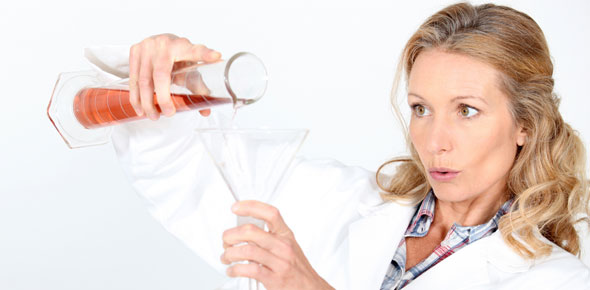# Chemical Reaction Types / Reaction Rates

25 Questions | Attempts: 183Settings• 1.
The process in which one or more substances change to make one or more new substances is called?
• A.

Chemical change

• B.

Chemical reaction

• C.

Chemical process

• D.

Chemical explosion

• 2.
How can you tell when a chemical reaction is taking place? (check all that apply)
• A.

A gas forms

• B.

Substance turns to a liquid

• C.

Energy is given off

• D.

There is a color change

• 3.
Is the chemical equation balanced?                            Na        +       Cl2   ------------------->    NaCl
• A.

Yes

• B.

No

• 4.
Is the chemical equation balanced?                    3Mg      +     N2 ------------->   Mg3N2
• A.

Yes

• B.

No

• 5.
Which of the following types of reactions results in a single product?
• A.

A synthesis reaction

• B.

A decomposition reaction

• C.

A combustion reaction

• D.

A single replacement reaction

• E.

A double replacement reaction

• 6.
Which of the following is NOT an observable clue that a chemical reaction has occurred?
• A.

Release of a gas

• B.

Odor change

• C.

Precipitate formation

• D.

Mixing two reactants

• 7.
The __________________________________________ says that mass cannot be created or destroyed.
• 8.
CO2(g)  +  H2O(l)  →     H2CO3(aq)
• A.

Synthesis

• B.

Decomposition

• C.

Single replacement

• D.

Double replacement

• 9.
2 Na(s)  +  Cl2(g)  →    2NaCl(s)
• A.

Synthesis

• B.

Decomposition

• C.

Single replacement

• D.

Double replacement

• 10.
Cl2(g)  +  2NaBr(aq)  →    2NaCl(aq)  +  Br2(l)
• A.

Synthesis

• B.

Decomposition

• C.

Single replacement

• D.

Double replacement

• 11.
2NaCl(l)  →    2Na(s)  +  Cl2(g)
• A.

Synthesis

• B.

Decomposition

• C.

Single replacement

• D.

Double replacement

• 12.
Zn(s)  +  2HCl(aq)  →    ZnCl2(aq)  +  H2(g)
• A.

Synthesis

• B.

Decomposition

• C.

Single replacement

• D.

Double replacement

• 13.
2KClO3(s)  →    2KCl(s)  +  3O2(g)
• A.

Synthesis

• B.

Decomposition

• C.

Single replacement

• D.

Double replacement

• 14.
CaCO3(s)  →    CaO(s)  +  CO2(g)
• A.

Synthesis

• B.

Decomposition

• C.

Single replacement

• D.

Double replacement

• 15.
2Mg(s)  +  O2(g)  →    2MgO(s)
• A.

Synthesis

• B.

Decomposition

• C.

Single replacement

• D.

Double replacement

• 16.
Ba(NO3)2(aq) + K2SO4(aq)    -->   BaSO4(s) + 2KNO3(aq)
• A.

Synthesis

• B.

Decomposition

• C.

Single replacement

• D.

Double replacement

• 17.
Fe(s)  +  CuSO4(aq)  →    FeSO4(aq)  +  Cu(s)
• A.

Synthesis

• B.

Decomposition

• C.

Single replacement

• D.

Double replacement

• 18.
C(s)  +  O2(g)  →    CO2(g)
• A.

Synthesis

• B.

Decomposition

• C.

Single replacement

• D.

Double replacement

• 19.
2P(s)  +  3Cl2(g)  →    2PCl3(g)
• A.

Synthesis

• B.

Decomposition

• C.

Single replacement

• D.

Double replacement

• 20.
H2SO4  →    H2O(l)  +  SO3(g)
• A.

Synthesis

• B.

Decomposition

• C.

Single replacement

• D.

Double replacement

• 21.
Ca(OH)2(s)  →    CaO(s)  +  H2O(g)
• A.

Synthesis

• B.

Decomposition

• C.

Single replacement

• D.

Double replacement

• 22.
Which statement is true?
• A.

The higher the temperature the slower the reaction

• B.

The lower the temperature the faster the reaction

• C.

The higher the concentration the slower the reaction

• D.

The higher the concentration the faster the reaction.

• 23.
The breaking down of a compound into simpler substances is
• A.

Displacement

• B.

Synthesis

• C.

Decomposition

• D.

Endothermic

• 24.
What coefficients would balance the following equation? Sr + H2O → Sr(OH)2 + H2
• A.

1, 4, 2, 2

• B.

2, 4, 2, 4

• C.

1, 2, 1, 1

• D.

• 25.
Which has the greater surface area?
• A.

A sugar cube (one teaspoon if measured)

• B.

A teaspoon of powdered sugar

• C.

They both have the same surface area.

• D.

There is no way to answer this question.

## Related TopicsBack to top
×

Wait!
Here's an interesting quiz for you.